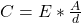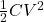## You slide a slab of dielectric between the plates of a parallel-plate capacitor. As you do this, the potential difference between the plates

Question

You slide a slab of dielectric between the plates of a parallel-plate capacitor. As you do this, the potential difference between the plates remains constant. What effect does adding the dielectric have on the energy stored in the capacitor?

in progress 0
6 months 2021-09-05T04:06:59+00:00 1 Answers 15 views 0

## Answers ( )

The energy stored by the capacitor is increased when a dielectric is added, with voltage or potential difference staying the same.

Explanation:

The capacitance of a parallel-plate capacitor will increase as a dielectric is added between the plates. This is according to the following equation for capacitance:Here, C is the capacitance, E is the dielectric constant, A is the area, and d is the distance between the plates.

Since the value of E is 1 or higher, the capacitance will increase once the dielectric is added.

The energy of a capacitor is found from the following equation:

Energy =here C is the capacitance, and V is the voltage.

Since the voltage stays constant, while the capacitance increases in the energy equation, the overall energy stored by the capacitor also increases.